# Common AC Current Sampling Circuits Lists and Design

In order to monitor a certain parameter in an electronic circuit, we need to be able to collect the current parameter in real-time, but we do not need to take all the data. At this time, we need to use the current sampling method to detect the current size. In practical applications, there are many sampling elements that can be used to measure the load current. The circuit composed of these sampling elements is the sampling circuit. Next, we will list several common AC current sampling circuits.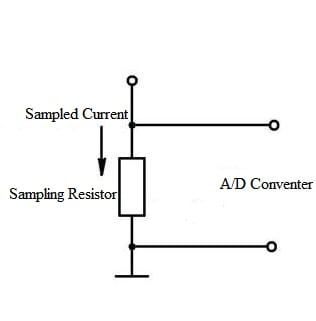Figure 1. Simple Current Sampling Circuit

#### Circuit 1

a. Current Conversion Circuit
The below picture shows the current conversion circuit, its performance is stable and reliable, and it is easy to install. How to choose the resistor R is relatively simple, you can count the required resistance according to the current required by the actual circuit.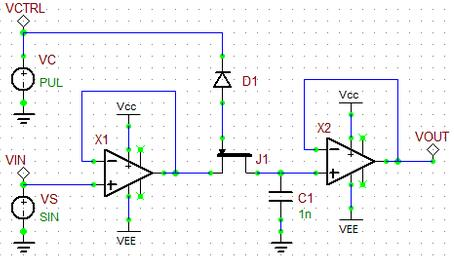Figure 2. AC Signal Sampling Circuit

In some cases, the Hall current sensor DT50-P will be used in circuits to monitor the current.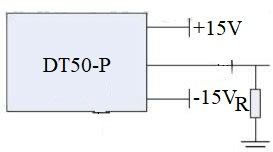Figure 3. Current Conversion Circuit

b. Filter compensation circuit
Since the detection point of voltage and current is the same point where STATCOM is connected to the power grid, its harmonic interference is a relatively large filter compensation circuit. Then the three-phase voltage and current must enter the filter compensation circuit after passing through their respective conversion circuits, including two parts: RC filtering and phase compensation.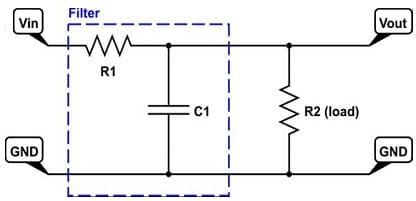Figure 4. RC Filtering Circuit

#### Circuit 2

a. Signal Amplifier Circuit
The AC signal amplifier circuit is shown in the figure. The transformer used in this design is SCT254AZ, which is a milliamp precision instrument. The rated input current is 5A, the rated output current is 2.5mA, the linear range is 0~20A, and the nonlinearity is <0.1%. After the phase shift is compensated less than 5, since the current output by the current transformer is good, there is no need to add a resistor R1.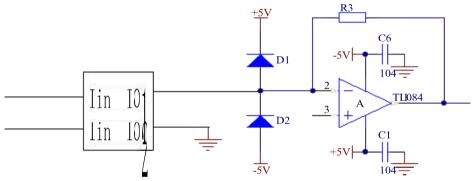Figure 5. Current Signal Amplifier Circuit

b. Second-order Filter Circuit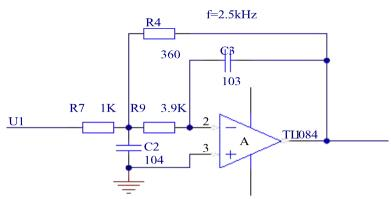Figure 6. Second-order Filter Circuit

The figure shows the second-order filter circuit, the cut-off frequency is 2.5KHz.

c. Unipolar Conversion Circuit
Because the A/D of the DSP used in the design requires the input signal to be 0~3.3V for sampling, the signal voltage connected to its pin cannot exceed 3.3V, so the bipolar number given by the amplifier circuit must do further processing. The unipolar conversion circuit is shown in the following figure: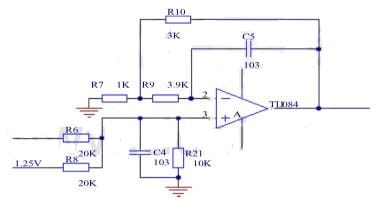Figure 7. Unipolar Conversion Circuit

#### Circuit 3

The phase current detection circuit is shown below, which uses an op-amp plus a voltage follower (isolation) for impedance matching before the A/D inlet. A clamping diode is used at the A/D inlet to prevent the input voltage from going out of bounds. The current signal from the current transformer of the detection channel converts the AC signal into a unipolar voltage signal of 0~3.3V after voltage translation and is connected to the A/D channel pin of the DSP.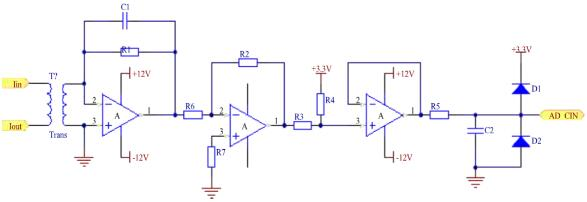Figure 8. Phase Current Detection Circuit

#### Circuit 4

The Hall current sensor uses the AC current of -100~100mA as the output signal, and the A/D input range of TMS320F2812 is 0~3V. So a suitable conditioning circuit must be added to meet the A/D input requirements. The AC current conditioning circuit is shown in the figure. The first part is filtered by the capacitor C4 and then flows through the sampling resistor ruler to convert the current signal into a voltage signal. The third part is the clamp limiter circuit to ensure that the output voltage signal is 0~3V, which meets the A/D input signal range of TMS320F2812.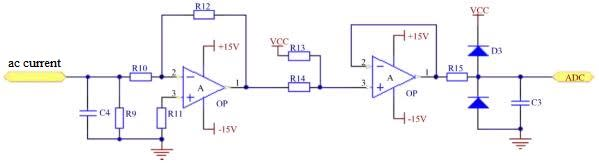Figure 9. Signal Sampling Circuit (ac current)

#### Circuit 5

Current acquisition TA014-2K precision AC current transformer, its rated input is 5A and rated output is 2.5A, operating frequency range is 20Hz~20kHz, the phase shift is less than 5', linear range is greater than 10A, and nonlinearity is less than 0.1%. In terms of these conditions, an ideal AC current detection device is made. The following figure shows the schematic diagram of the current acquisition circuit.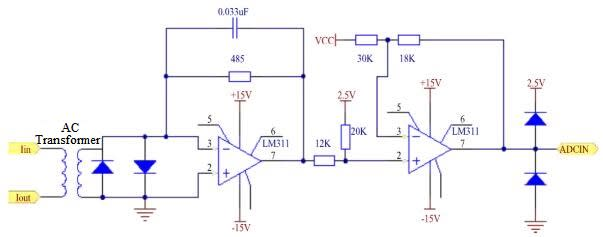Figure 10. AC Circuit Sampling Circuit

Since the A/D input signal range of the DSP is 0~3.3V, the current signal measured by the current transformer is converted into an AC signal of -1.5V~1.5V, so it is shifted by 1.5V.

#### AC Current Sampling Circuit Design

According to the common AC current sampling circuit design above, the sensor selects the Hall current sensor DT50-P. Choosing the resistance value of resistor R8 depends on the input requirements of the AC signal conditioning circuit. The conditioning circuit needs to input an AC voltage signal of -5V~5V, then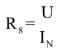The resistance value of R8 can be determined: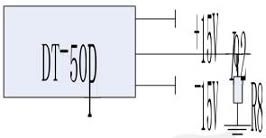Figure 11. Current Conversion Circuit

In the figure, the actual value of the current is converted into a 5V voltage signal (Io2) after passing through the Hall current sensor and the sampling resistor, and the 5V signal is reversed. Io2 is filtered first. After filtering out noise interference, the selection of filter resistors and capacitors should meet the requirement that the time constant is less than 1ms. Therefore, the optional R9 is 100 kΩ and C6 is 220pF, and converted to -3.3V~3.3V signal. After 3.3V level boost circuit and average care, the input of the voltage follower is a 0~3.3V unipolar signal, and the resistance values of R13 and R14 are only the same. Here, a resistor with 10 kiloohms is selected, which is safe and meets the requirements. Finally, through the limiting of two series diodes, the signal input to the DSP is ensured to be 0~3.3V, so as to ensure that the DSP will not be burned. And the model is shown in the figure below: R15=1KΩ. The principle of the current sampling method of each phase is the same.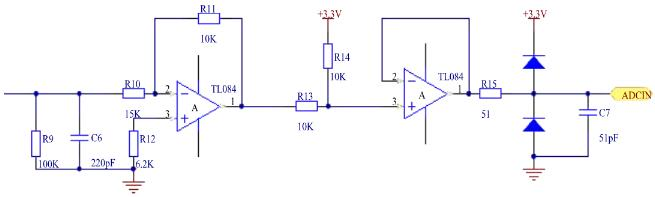Figure 12. Current Conditioning Circuit